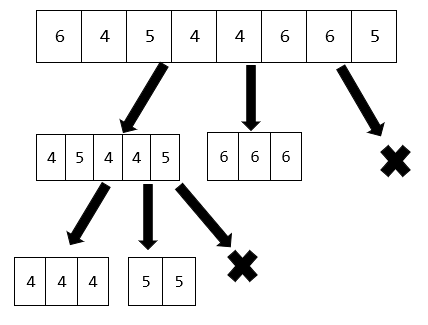×

Search anything:

# 3 Way Partitioning Quick Sort

#### Algorithms Sorting Algorithms quick sortGet this book -> Problems on Array: For Interviews and Competitive Programming

In this article, we have explored 3 Way Partitioning Quick Sort in depth. This is relatively faster than 2 way Quick Sort (Normal version) in practical applications.

1. Introduction to Quick Sort
2. 3 Way Partitioning Quick SortImplementation of 3 way partitioning of Quick SortComparison with 2 way partitioning quick sort

Pre-requisites:

## Introduction to Quick Sort

The Quick sort algorithm is a sorting algorithm based on the divide and conquer strategy. Here, an element (usually called a pivot) is picked, and elements are arranged such that the pivot element acquires its position in the sorted array. The sub-arrays consisting of elements before and after this pivot element are then similarly split into partitions. This process of partitioning the array around pivots continues until the array gets sorted.

In conventional quick sort, 2 way partitioning is performed. Here, the array is split into two sub-arrays (partitions): one consisting of elements less than or equal to the pivot element, and the other consisting of elements greater than the pivot element.

We shall first discuss another type of quick sort, called Three way partitioning quick sort where 3 partitions are formed instead of just 2.

## 3 Way Partitioning Quick Sort

The functioning of the 3 way quick sort is similar to that of the conventional quick sort. Here, a pivot element is selected, and elements are rearranged and three partitions are formed as follows:

1. Elements which are less than the pivot element.
2. Elements equal to the pivot element.
3. Elements greater than the pivot element.

Then, the procedure is repeated on the first and the third partitions. Note that the 2nd sub-array is already sorted and has taken its correct position in the final sorted array. This process of partitioning the array into sub-arrays continues until each sub-array has either one element or a consists of equal elements.

Tracing an example

We shall try sorting the array [6, 4, 5, 4, 4, 6, 6, 5] using this algorithm.

• First, the three way partitioning is performed on the given array, with 4 as the pivot element, and we obtain the partitions as [4, 5, 4, 4, 5], [6, 6, 6], and [].
• Now, we call the three way partitioning on [4, 5, 4, 4, 5] with 4 as the pivot element and obtain the partitions as [4, 4, 4], [5, 5] and [].
• Now, we call the partitioning algorithm again on [4, 4, 4], and obtain the partitions as [], [4, 4, 4] and []. Hence we are done.
• We finally combine the sub-arrays and hence obtain [4, 4, 4, 5, 5, 6, 6, 6].

The overall solution can be summarized as follows:## Implementation of 3 way partitioning of Quick Sort

We now give one way of implementing the solution using C++.

``````#include<iostream>
using namespace std;

void TWP(int arr[], int m, int n, int &begin, int &end)
{
int pivot = arr[m];
begin = m, end = n;

for(int i = begin + 1 ; i <= end; i++)
{
if(arr[i] < pivot)
{
swap(arr[i], arr[begin]);
begin++;
}
else if(arr[i] > pivot)
{
swap(arr[i], arr[end]);
end--;
i--;
}
}
}

void quicksort(int arr[], int m, int n)
{
if(m>=n)
{
return;
}
int begin, end;
TWP(arr, m, n, begin, end);
quicksort(arr, m, --begin);
quicksort(arr, ++end, n);
}

int main()
{
int arr[] = {3, 9, 7, 7, 5, 5, 7, 3, 7, 9};
int n = 10;
quicksort(arr, 0, n-1);
for(int i = 0; i < n; i++)
cout<<arr[i]<<endl;
}
``````

On average, we can see that the number of recursive calls will be of order log n, and since each function call consists of a loop, the average time complexity of this solution is given by O(nlogn). Since each recursion takes O(1) space, and there are log n recursions on average, the average space complexity is given by O(log n).

The worst case of this problem would be when the least or the highest element is considered as pivot, in which case the recursive call would be called on an array with n-1 elements. Hence, the worst case time complexity of this solution is O(n2).

## Comparison with 2 way partitioning quick sort

The average time complexity of the 2 way partitioning quick sort is given by O(nlog2n), which is slightly greater than that of the 3 way partitioning quick sort (O(nlog3n)).

This is not the only advantage 3 way quicksort has over the conventional method. It also performs with an O(1) in the trivial case where all the elements in the given array happen to be equal. For this case, the 2 way partitioning quick sort functions with a time complexity of O(n2).

One can conclude that the 3 way partitioning quicksort would be better in case there are multiple repeated elements in the array, while in case of an array with distinct elements, both methods function with approximately the same time complexity.

With this article at OpenGenus, you must have the complete idea of 3 Way Partitioning Quick Sort.#### Aravind Mohandas

Aravind Mohandas is a Master's student at Indian Institute of Technology, Ropar with B.Sc in Computer Science from Christ University, Bangalore. He is an intern at OpenGenus.

3 Way Partitioning Quick Sort